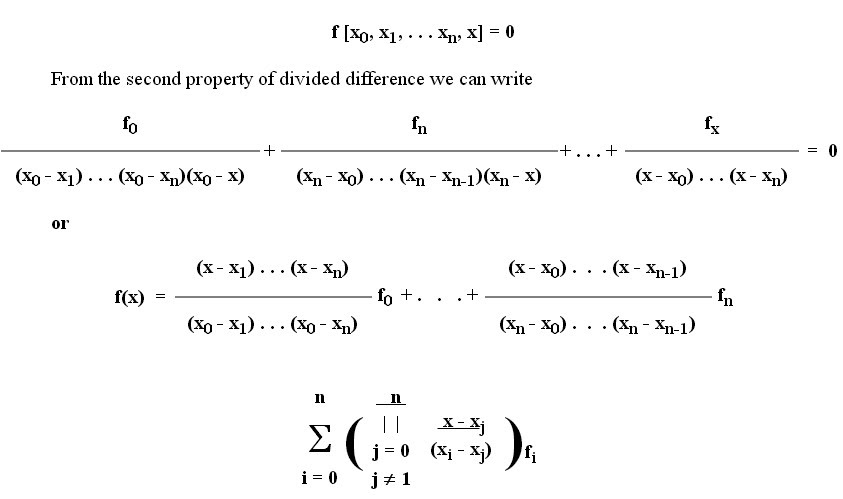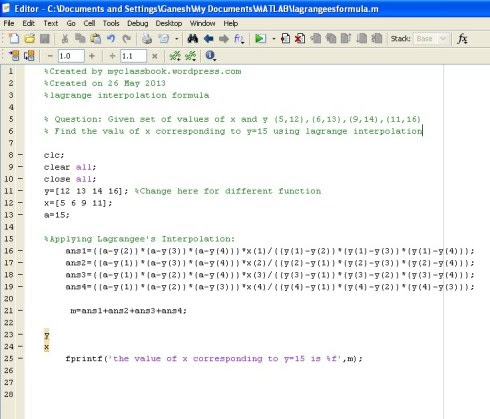# Lagrange Interpolation With MATLAB Program Example

Lagrange's Interpolation Formula is used to determine the value of any function f(x), which is known at discrete points. That is if we have any function with its value at different points such as, at x=0, 1, 2... So using Lagrange's Interpolation Formula, we can determine the value of that function at any point.

## Derivation

We can derive the Lagrange's Interpolation formula by using Newton's divided difference formula. If f(x) is approximated with a Nth degree polynomial then the Nth divided difference of f(x) constant and (N+1)th divided difference is zero. That isas we know Lagrange's interpolation is a Nth degree polynomial approximation to f(x) and the Nth degree polynomial passing through (N+1) points is unique hence the Lagrange's and Newton's divided difference approximations are one and the same. However, Lagrange's interpolation formula is very useful for the computer programming while Newton's difference formula is convenient for the hand calculations.

Question: Given set of values of x and y (5,12),(6,13),(9,14),(11,16)
Find the value of x corresponding to y=15 using lagrange interpolation

## Solution

Tabular the given data:

y:  12  13  14  16

x:   5    6    9    11

Applying lagrange interpolation formula,

x(y)=(y-13)(y-14)(y-16)*5/(12-13)(12-14)(12-16)+

(y-12)(y-14)(y-16)*6/(13-12)(13-14)(13-16)+

(y-12)(y-13)(y-16)*9/(14-12)(14-13)(14-16)+

(y-12)(y-13)(y-14)*11/(16-12)(16-13)(16-14).

By putting y=15 we get x(15)=11.5

## MATLAB Code for Lagrange Interpolation Formula

`%Created by myclassbook.org%Created on 26 May 2013%lagrange interpolation formula% Question: Given set of values of x and y (5,12),(6,13),(9,14),(11,16)% Find the value of x corresponding to y=15 using lagrange interpolationclc;  clear all;  close all;  y=[12 13 14 16]; %Change here for different function  x=[5 6 9 11];  a=15;%Applying Lagrange's Interpolation:  ans1=((a-y(2))*(a-y(3))*(a-y(4)))*x(1)/((y(1)-y(2))*(y(1)-y(3))*(y(1)-y(4)));  ans2=((a-y(1))*(a-y(3))*(a-y(4)))*x(2)/((y(2)-y(1))*(y(2)-y(3))*(y(2)-y(4)));  ans3=((a-y(1))*(a-y(2))*(a-y(4)))*x(3)/((y(3)-y(1))*(y(3)-y(2))*(y(3)-y(4)));  ans4=((a-y(1))*(a-y(2))*(a-y(3)))*x(4)/((y(4)-y(1))*(y(4)-y(2))*(y(4)-y(3))); m=ans1+ans2+ans3+ans4;yxfprintf('the value of x corresponding to y=15 is %f',m);`

## Image Format

[caption id="" align="aligncenter" width="490"]MATLAB code for Lagrange interpolation[/caption]

1.2.